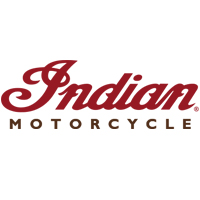# Indian Motorcycle Quiz## Think you’re a car expert? Prove it! Take our fun Indian Motorcycle Quiz and put your knowledge to the test!

• You will be given 20 seconds to answer each question.
• The quicker you correctly answer the question, the more points you receive.
• When you finish, you will be able to review your results.

Accuracy:

\${ correct_answers }\$ / \${ questions.length }\$
Avg. User Score \${ average_score }\$%

Speed Points:

\${ calculate_avg_speedpoints.total_earned_speedpoints_percentage }\$%
\${ calculate_avg_speedpoints.total_earned_speedpoints }\$ / \${ calculate_avg_speedpoints.total_possible_speedpoints }\$
Avg. User Score \${ average_speed_points }\$%
Total Score:   Weighted Avg: 80% Accuracy & 20% Speed
\${ calculate_avg_speedpoints.total_score }\$%
\${ calculate_avg_speedpoints.total_score }\$ / 100
Avg. User Score \${ average_total_score }\$%
Top Score \${ top_score }\$%
Speed Value: \${ speedPoints }\$ pts \${ timerSecondsRemainingInt }\$ sec